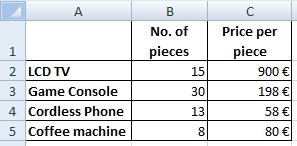# How to calculate the Grand Total using SUMPRODUCT

SUMPRODUCT is very useful Excel function. It helps us to find the grand total of the table without need auxiliary calculations. This function multiplies corresponding items in arrays and then it sums their results. In math it’s called scalar product or dot product.

## Example 1

There is a list of products. We want to find out the total price of all products.The formula without SUMPRODUCT:
=B2*C2+B3*C3+B4*C4+B5*C5

The formula with SUMPRODUCT:
=SUMPRODUCT(B2:B5,C2:C5)

Result:  20834 €

## Example 2

Let’s have 2 tables. The first contains number of pieces in 2 stores. The second contains price per piece in this 2 stores. We want to know the total price of all products in both stores together.The formula without SUMPRODUCT:
=B3*G3+B4*G4+B5*G5+B6*G6+C3*H3+C4*H4+C5*H5+C6*H6

The formula with 2 SUMPRODUCT functions:
=SUMPRODUCT(B3:B6,G3:G6)+SUMPRODUCT(C3:C6,H3:H6)

The formula with one SUMPRODUCT function:
=SUMPRODUCT(B3:C6,G3:H6)

Result: 37954 €

1.